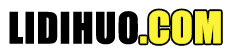# AI 统一

P(x，y).........(i)
P(a，f(z)).........(ii)

## 统一算法:

```Step. 1: If Ψ1 or Ψ2 is a variable or constant, then:
a) If Ψ1 or Ψ2 are identical, then return NIL.
b) Else if Ψ1is a variable,
a. then if Ψ1 occurs in Ψ2, then return FAILURE
b. Else return { (Ψ2/ Ψ1)}.
c) Else if Ψ2 is a variable,
a. If Ψ2 occurs in Ψ1 then return FAILURE,
b. Else return {( Ψ1/ Ψ2)}.
d) Else return FAILURE.
Step.2: If the initial Predicate symbol in Ψ1 and Ψ2 are not same, then return FAILURE.
Step. 3: IF Ψ1 and Ψ2 have a different number of arguments, then return FAILURE.
Step. 4: Set Substitution set(SUBST) to NIL.
Step. 5: For i=1 to the number of elements in Ψ1.
a) Call Unify function with the ith element of Ψ1 and ith element of Ψ2, and put the result into S.
b) If S = failure then returns Failure
c) If S ≠ NIL then do,
a. Apply S to the remainder of both L1 and L2.
b. SUBST= APPEND(S, SUBST).
Step.6: Return SUBST. ```

## 算法的实现

Step.1: 将替换集初始化为空。
Step .2: 以递归方式统一原子句子:

1、在{p(f(a)，g(Y))和p(X，X)}的MGU中查找
Sol: S 0 =>此处，Ψ 1 = p(f(a)，g(Y))，Ψ 2 = p(X，X)
SUBSTθ= {f( a)/X}
s1 =>Ψ 1 = p(f(a)，g(Y))，Ψ 2 = p(f(a )，f(a))
SUBSTθ= {f(a)/g(y)}，统一失败。

2、找出{p(b，X，f(g(Z)))和p(Z，f(Y)，f(Y))}的MGU

S 0 => {p(b，X，f(g(Z))); p(Z，f(Y)，f(Y))}
SUBSTθ= {b/Z}
S 1 => {p(b，X ，f(g(b))); p(b，f(Y)，f(Y))}
SUBSTθ= {f(Y)/X}
S 2 => {p( b，f(Y)，f(g(b))); p(b，f(Y)，f(Y))}
SUBSTθ= {g(b)/Y}
S 2 => {p( b，f(g(b))，f(g(b)); p(b，f(g(b))，f(g(b))} 已成功统一。

3、找到{p(X，X),和p的MGU(Z，f(Z))}

S 0 => {p(X，X)，p(Z，f(Z))}
SUBSTθ= {X/Z}
s1 => {p(Z，Z)，p(Z，f(Z))}
SUBSTθ= {f(Z)/Z}，统一失败

4、找到UNIFY的MGU(prime(11),prime(y))

S 0 => {prime(11)，prime(y)}
SUBSTθ= {11/y}
S 1 => {prime(11)，prime(11)}，已成功统一。

5、找到Q(a，g(x，a)，f(y))，Q的MGU(a，g(f(b)，a)，x)}

S 0 => {Q( a，g(x，a)，f(y)); Q(a，g(f(b)，a)，x)}
SUBSTθ= {f(b)/x}
S 1 => {Q(a， g(f(b)，a)，f(y)); Q(a，g(f(b)，a)，f(b))}
SUBSTθ= {b/y}
S 1 => { Q(a，g(f(b)，a)，f(b)); Q(a，g(f(b)，a)，f(b))}，已成功统一。

6、 UNIFY(knows(Richard，x)knows(Richard，John))
Ψ1 = knows(Richard, x), and Ψ2 = knows(Richard, John)
S0 => { knows(Richard, x); knows(Richard, John)}
SUBST θ= {John/x}
S1 => { knows(Richard, John); knows(Richard, John)},成功统一。

"内容整理不易，点下广告也是鼓励～"

Copyright © 2020 立地货 All Rights Reserved.